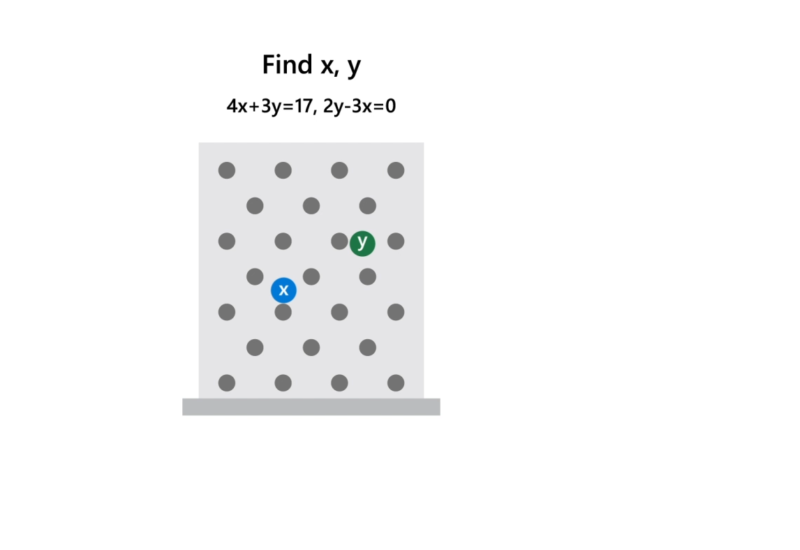# Microsoft Research Blog

The Microsoft Research blog provides in-depth views and perspectives from our researchers, scientists and engineers, plus information about noteworthy events and conferences, scholarships, and fellowships designed for academic and scientific communities.

## Next-generation architectures bridge gap between neural and symbolic representations with neural symbols

December 12, 2019 | By Paul Smolensky, Partner Researcher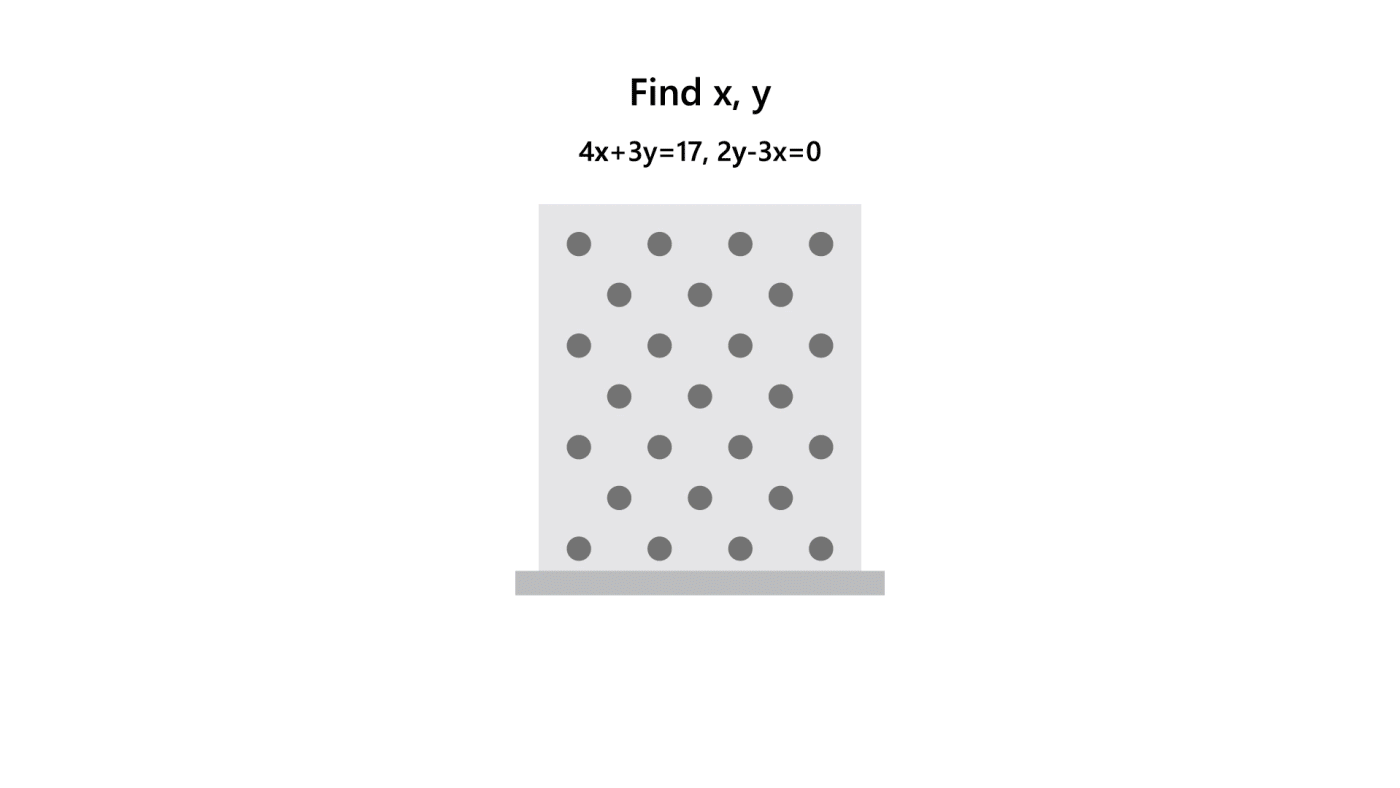In both language and mathematics, symbols and their mutual relationships play a central role. The equation x = 1/y asserts the symbols x and y—that is, what they stand for—are related reciprocally; Kim saw the movie asserts that Kim and the movie are perceiver and stimulus. People are extremely adept with the symbols of language and, with training, become adept with the symbols of mathematics. For many decades, cognitive science explained these human abilities by assuming the presence of symbols in the mind, and AI researchers emulated these abilities by building complex machines for processing symbols. But how could discrete symbols, and the abstract relations between them, be manifested in the neural networks of the brain? The dramatic and recent progress in AI has, in fact, been fueled by replacing the previous symbol-based systems with fundamentally different artificial neural network–based models inspired by the brain. In this new generation of AI systems, is there any place for symbols?

At Microsoft Research AI, and in other AI labs, there has been considerable advancement in the development of new types of intelligent systems: neurosymbolic AI models. In neurosymbolic AI, symbol processing and neural network learning collaborate. Using a unique neurosymbolic approach that borrows a mathematical theory of how the brain can encode and process symbols, we at Microsoft Research are building new AI architectures in which neural networks learn to encode and internally process symbols—neural symbols. Neural symbols, technically known as Tensor Product Representations (TPRs), are patterns of activation distributed over large collections of neurons. Unlike those of standard neural network models, these patterns have a special internal structure that for the first time allows neural computation to process them the way symbols were processed in traditional symbol-based AI systems.

Two of these new neural architectures—the Tensor-Product Transformer (TP-Transformer) and Tensor Products for Natural- to Formal-Language mapping (TP-N2F)—have set a new state of the art for AI systems solving math and programming problems stated in natural language. Because these models incorporate neural symbols, they’re not completely opaque, unlike nearly all current AI systems. We can inspect the learned symbols and their mutual relations to begin to understand how the models achieve their remarkable results. We’re presenting both architectures during workshops at the 33rd Conference on Neural Information Processing Systems (NeurIPS).

### Solving math problems

The recently released Mathematics Dataset contains millions of problems across domains, including algebra, calculus, probability, and number theory, up to the high school level and beyond. Each problem is stated in English and comes with a solution in English. To count as correct, the answer from a model must match character for character the dataset’s answer.

The TP-Transformer model—the powerful Transformer architecture enhanced with neural symbols—raised the state-of-the-art overall success level on the dataset from 76 percent to 84 percent. Relative to the previous state of the art, the TP-Transformer outperforms the previous model or performs perfectly in all but one of the 56 mathematical subareas distinguished in the dataset. The following examples of test problems (not seen during learning) from the dataset, along with the (correct) answers generated by our TP-Transformer model, demonstrate the model’s capacity to perform multistep inference in number theory, algebra, and calculus:

Suppose 0 = 2*a + 3*a − 150. Let p = 106 − 101. Suppose −3*b + w + 544 = 3*w, −p*b − 5*w = −910. What is the greatest common factor of b and a?

30

Let q(l) = 33*l. Let a(y) = −y**2 + 2*y − 2. Let p be a(1). Let d be q(p). Let n = 38 + d. Solve −5*v − 11 = −3*c − 0*v, −4*c = n*v + 32 for c.

−3

Let r(g) be the second derivative of 2*g**3/3 − 21*g**2/2 + 10*g. Let z be r(7). Factor −z*s + 6 − 9*s**2 + 0*s + 6*s**2.

−(s + 3)*(3*s − 2)

The MathQA dataset also consists of a variety of math word problems, but rather than producing answers in English, here the goal is to produce a simple solution program: a sequence of steps, each a primitive operation, consisting of an operator together with the arguments it applies to. Again, to count as correct, a model’s answer must match symbol for symbol the answer provided in the dataset. TP-N2F—a general architecture for taking descriptions in English as inputs and producing outputs in formal languages, such as logic—raised the state-of-the-art success rate from 59 percent to 72 percent.

The following examples of test problems (not seen during learning) and the (correct) answer produced by TP-N2F demonstrate how much English text the model can read and “understand” and how long a program the model can generate:

this year , mbb consulting fired 6 % of its employees and left remaining employee salaries unchanged . sally , a first – year post – mba consultant , noticed that the average ( arithmetic mean ) of employee salaries at mbb was 10 % more after the employee headcount reduction than before . the total salary pool allocated to employees after headcount reduction is what percent of that before the headcount reduction ?

(multiply n1 const_100) (subtract const_100 n0) (add #0 const_100) (add #1 const_4) (multiply #2 #3) (divide #4 #0)

a high school has 360 students 1 / 2 attend the arithmetic club , 5 / 8 attend the biology club and 3 / 4 attend the chemistry club . 3 / 8 attend all 3 clubs . if every student attends at least one club how many students attend exactly 2 clubs .

(multiply n0 n1) (multiply n0 n3) (multiply n0 n5) (divide #0 n2) (divide #1 n4) (divide #2 n6) (divide #2 n4) (add #3 #4) (multiply n2 #6) (add #7 #5) (subtract #9 #8) (subtract #10 n0)

TP-N2F was also applied to another problem, generating Lisp programs from English descriptions: the AlgoLisp dataset. Again, the new neurosymbolic model substantially improved the state of the art, from 77 percent to 93 percent. Here is an example of its correct performance, a solution consisting of 55 Lisp commands:

given numbers a , b , c and e , let d be c , reverse digits in d , let a and the number in the range from 1 to b inclusive that has the maximum value when its digits are reversed be the coordinates of one end and d and e be the coordinates of another end of segment f , find the length of segment f squared

(digits c) (reverse #0) (* arg1 10) (+ #2 arg2) (lambda2 #3) (reduce #1 0 #4) (− a #5) (digits c) (reverse #7) (* arg1 10) (+ #9 arg2) (lambda2 #10) (reduce #8 0 #11) (− a #12) (* #6 #13) (+ b 1) (range 0 #15) (digits arg1) (reverse #17) (* arg1 10) (+ #19 arg2) (lambda2 #20) (reduce #18 0 #21) (digits arg2) (reverse #23) (* arg1 10) (+ #25 arg2) (lambda2 #26) (reduce #24 0 #27) (> #22 #28) (if #29 arg1 arg2) (lambda2 #30) (reduce #16 0 #31) (− #32 e) (+ b 1) (range 0 #34) (digits arg1) (reverse #36) (* arg1 10) (+ #38 arg2) (lambda2 #39) (reduce #37 0 #40) (digits arg2) (reverse #42) (* arg1 10) (+ #44 arg2) (lambda2 #45) (reduce #43 0 #46) (> #41 #47) (if #48 arg1 arg2) (lambda2 #49) (reduce #35 0 #50) (− #51 e) (* #33 #52) (+ #14 #53)

### Under the hood

With neurosymbolic models, we can peer inside and examine the neural symbols learned and the learned relations between them. In the TP-Transformer model, for example, we find that digits in the denominator of a fraction seek to fill one set of relations, while digits in the numerator seek different relations. When processing the input 2/5 + 3/7, we see that to form the ultimate encoding of the symbol 5, a query is issued that looks for a division operator for which 5 is in the denominator; that query matches the first slash.

These observations are just initial steps in the long process of decoding how the TP-Transformer model manages to perform so well on challenging math problems and to explain why it falls short when it does. But these interpretations of the learned relations between neural symbols are immediately evident, so the prospects are good for developing more sophisticated methods to achieve deep understanding of how these models work.

In the TP-N2F model of MathQA solution-program generation, we can examine the vectors that the model learns for encoding, for example, the operators. We find that general-purpose operators like add, negate, and log fall in one region of the vector space, while shape-specific geometric computations such as square_area, volume_cylinder, and surface_cube fall into a different region. At one boundary of the space are max, min; at another are factorial, choose (a complex function built from factorial). We begin to see how the model has laid out its learned relation space and can start to analyze how that structure supports its success on its task.

### The promise of a fundamental shift

Symbol processing has been a powerful theory of many of the abilities defining human intelligence, and Microsoft Research’s unique approach to neurosymbolic AI promises significant progress in diverse application areas—progress in creating AI systems that not only perform well, but that can also be understood. Such understanding helps us to explain why a model makes the mistakes it does and enables us to go into the bowels of the model and directly alter the activations of neurons to produce a desired change in behavior. This promises a fundamental shift in the way humans interact with AI models.

The TP-Transformer release package, which contains the pretrained models, the source code, and a README document describing step-by-step how to reproduce the results reported in the TP-Transformer paper, is available to the public on GitHub. A similar package for TP-N2F will also be made available on GitHub. We look forward to ongoing dialogue with the research community on the use of neural symbols in AI.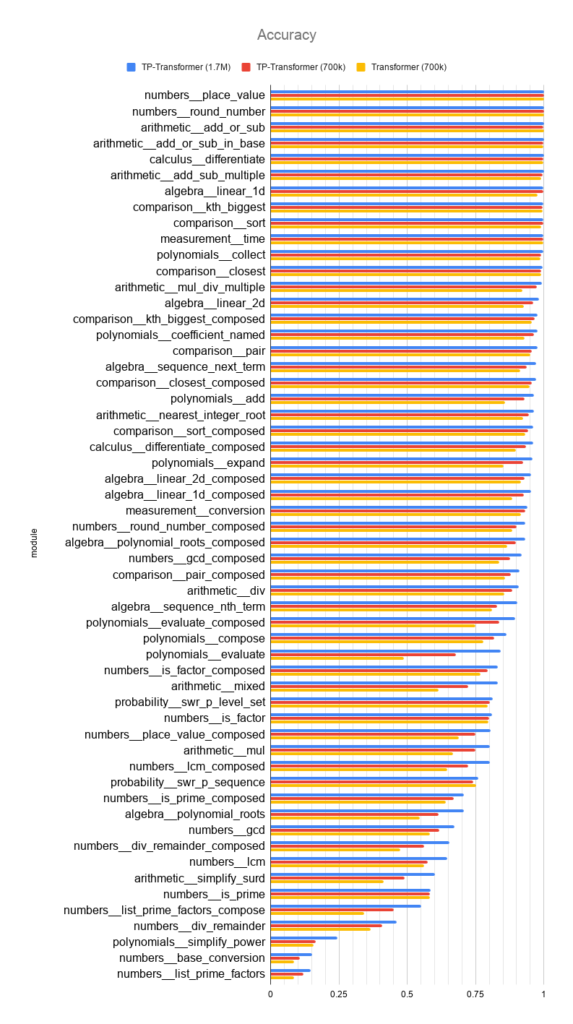The above figure shows the proportion of unseen problems answered correctly for each type of math problem in the Mathematics Dataset for the previous state-of-the-art model (yellow), for the TP-Transformer trained on the same quantity of data (red; 700K steps), and for the TP-Transformer trained on approximately 2.5 times as much data (blue; 1.7M steps). In all but one subarea, TP-Transformer—a neurosymbolic model—outperforms the previous model or performs perfectly.

## Up Next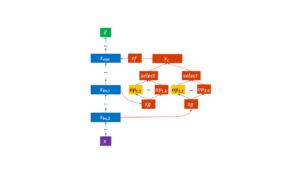Algorithms, Artificial intelligence, Computer vision

### Project Petridish: Efficient forward neural architecture search

Having experience in deep learning doesn’t hurt when it comes to the often mysterious, time- and cost-consuming process of hunting down an appropriate neural architecture. But truth be told, no one really knows what works the best on a new dataset and task. Relying on well-known, top-performing networks provides few guarantees in a space where […]Principal ResearcherArtificial intelligence

### ReDial: Recommendation dialogs for bridging the gap between chit-chat and goal-oriented chatbots

Chatbots come in many flavors, but most can be placed in one of two categories: goal-oriented chatbots and chit-chat chatbots. Goal-oriented chatbots behave like a natural language interface for function calls, where the chatbot asks for and confirms all required parameter values and then executes a function. The Cortana chat interface is a classic example […]Microsoft blog editor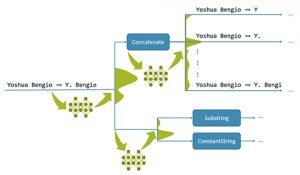Artificial intelligence, Programming languages and software engineering

### Neural-Guided Deductive Search: A best of both worlds approach to program synthesis

Program synthesis — automatically generating a program that satisfies a given specification — is a major challenge in AI. In addition to changing the way we design software, it has the potential to revolutionize task automation. End users without programming skills can easily provide input-output examples of the desired program behavior. The Flash Fill feature […]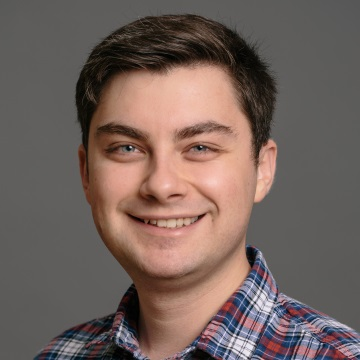Alex Polozov

Senior Researcher